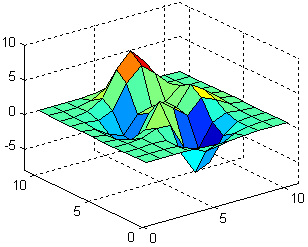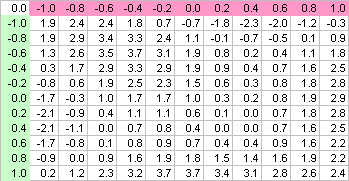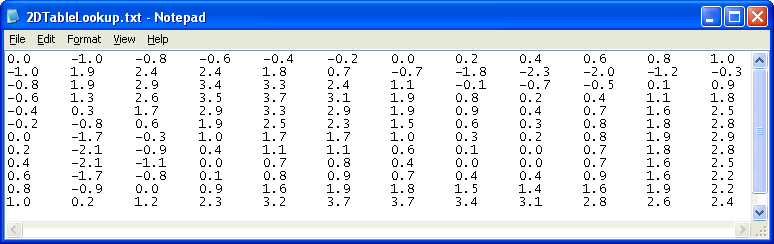﻿ 20-sim webhelp > Library > Signal > Block Diagram Non-Linear > function-2dtable

# function-2dtable

## Library

Signal\Block Diagram Non-Linear

## Use

Domains: Continuous. Size: 1-D. Kind: Block Diagrams.

## Description

This model uses a two-dimensional table with data points to calculate the output z = f(x,y) as a function of the input x and y. The output z is calculated using linear interpolation between the table data points.

 • The first row denotes the x-values and the first column denotes the y-values.
 • The input data of the first column and first row needs to be monotonically increasing.
 • Discontinuities are allowed, by providing the same input point twice in first row or first column of the table.
 • Values outside of the table range, are computed by linear extrapolation of the last two points.

A table must be stored as an ASCII (text) file and should consist rows and columns of data. The first row consists of the x-values first column consist of the y-values. The row-column pairs are the corresponding z-values. Values must be separated by a space or a tab. No comment or other text may be part of the table-file. The filename of the input file can be specified using the complete path (e.g. c:\data\data.tbl ). When no path is given, the file is assumed to be in the experiment directory.

## Example

Suppose we want to use the well known Matlab peaks function:This function can be put in a matrix form as:The first row and column denote the x-values and y-values and the other row-column pairs the corresponding peaks function values. This matrix can be entered in a text file:The model 2DTableLookup.emx (Examples\Signal Processing) shows how to use this text file as a 2-D table.

## Interface

 Inputs Description input1 input2 x-value y-value Outputs output z-value Parameters filename The filename of the table file.